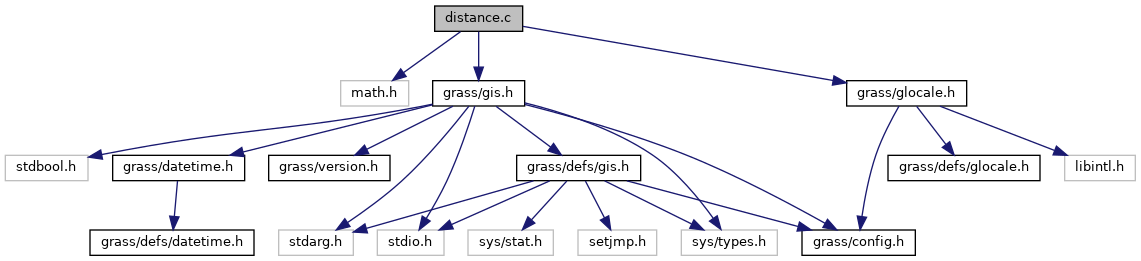GRASS GIS 7 Programmer's Manual  7.9.dev(2020)-547ded6c0
gis/distance.c File Reference

GIS Library - Distance calculation functions. More...

`#include <math.h>`
`#include <grass/gis.h>`
`#include <grass/glocale.h>`
Include dependency graph for gis/distance.c:Go to the source code of this file.

## Functions

int G_begin_distance_calculations (void)
Begin distance calculations. More...

double G_distance (double e1, double n1, double e2, double n2)
Returns distance in meters. More...

double G_distance_between_line_segments (double ax1, double ay1, double ax2, double ay2, double bx1, double by1, double bx2, double by2)
Returns distance between two line segments in meters. More...

double G_distance_point_to_line_segment (double xp, double yp, double x1, double y1, double x2, double y2)
Returns distance between a point and line segment in meters. More...

## Detailed Description

GIS Library - Distance calculation functions.

WARNING: this code is preliminary and may be changed, including calling sequences to any of the functions defined here.

(C) 2001-2009, 2011 by the GRASS Development Team

This program is free software under the GNU General Public License (>=v2). Read the file COPYING that comes with GRASS for details.

Definition in file gis/distance.c.

## ◆ G_begin_distance_calculations()

 int G_begin_distance_calculations ( void )

Begin distance calculations.

Initializes the distance calculations. It is used both for the planimetric and latitude-longitude projections.

Returns
0 if projection has no metrix (ie. imagery)
1 if projection is planimetric
2 if projection is latitude-longitude

Definition at line 42 of file gis/distance.c.

Referenced by Vect_line_geodesic_distance().

## ◆ G_distance()

 double G_distance ( double e1, double n1, double e2, double n2 )

Returns distance in meters.

This routine computes the distance, in meters, from x1,y1 to x2,y2. If the projection is latitude-longitude, this distance is measured along the geodesic. Two routines perform geodesic distance calculations.

Parameters
 e1,n1 east-north coordinates of first point e2,n2 east-north coordinates of second point
Returns
distance

Definition at line 75 of file gis/distance.c.

References G_geodesic_distance(), and PROJECTION_LL.

Referenced by Vect_line_geodesic_distance().

## ◆ G_distance_between_line_segments()

 double G_distance_between_line_segments ( double ax1, double ay1, double ax2, double ay2, double bx1, double by1, double bx2, double by2 )

Returns distance between two line segments in meters.

Parameters
 ax1,ay1,ax2,ay2 first segment bx1,by1,bx2,by2 second segment
Returns
distance value

Definition at line 91 of file gis/distance.c.

References G_intersect_line_segments(), and x.

## ◆ G_distance_point_to_line_segment()

 double G_distance_point_to_line_segment ( double xp, double yp, double x1, double y1, double x2, double y2 )

Returns distance between a point and line segment in meters.

Parameters
 xp,yp point coordinates x1,y1 segment point coordinates x2,y2 segment point coordinates
Returns
distance

Definition at line 120 of file gis/distance.c.